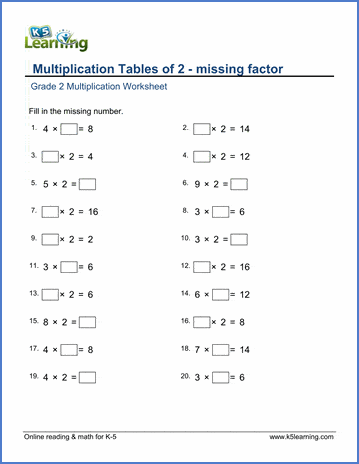# Multiplication tables of 2 - missing factor

## Worksheets: Multiplication tables of 2 with missing factors

Below are six versions of our grade 2 math worksheet on multiplication tables of 2; students must find the missing factors. These exercises reinforce recall of basic multiplication facts.  These worksheets are pdf files.## More multiplication worksheets

Find all of our multiplication worksheets, from basic multiplication facts to multiplying multi-digit whole numbers in columns.

## What is K5?

K5 Learning offers reading and math worksheets, workbooks and an online reading and math program for kids in kindergarten to grade 5.  We help your children build good study habits and excel in school.